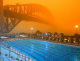# Math Is Fun Forum

Discussion about math, puzzles, games and fun.   Useful symbols: ÷ × ½ √ ∞ ≠ ≤ ≥ ≈ ⇒ ± ∈ Δ θ ∴ ∑ ∫  π  -¹ ² ³ °

You are not logged in.

## #1 2022-12-13 10:33:30

Keckman
Member
From: Helsinki Finland
Registered: 2022-12-13
Posts: 14
Website

### Very good approximation of pi by rational number

355/113=3.141592920353982300884955752212389380530973451327433628318584070796460176991150...
is a 'terrible good' approximation of pi! There is six number in divide operation and you get seven right numbers of pi. But is there even better ones? Let's examine it with Pascal program:

``````program approximation;
uses bigdecimalmath;
type stri = array[1..80] of char;
var a,b,approx:BigDecimal;
right,i,best,len:LongInt;
str1,str2,str3:stri;
piStr:String;
begin
piStr:='3.141592653589793238462643383279502884197169399375';
a:=1;
while a<=StrToBigDecimal('1000000000000') do
begin
str1:=BigDecimalToStr(a);
Right:=0;
b:=round(StrToBigDecimal(piStr)*a);
str2:=BigDecimalToStr(b);
approx:=divide(b,a,9999);
str3:=BigDecimalToStr(approx);
i:=1;
while(str3[i]=piStr[i]) do
begin
Right:=Right+1;
i:=i+1;
end;
len:=Length(BigDecimalToStr(a))+Length(BigDecimalToStr(b));
if (right-1)>len then
begin
writeln(str1:6,' ',str2:6,'  ',len,'  ',str3:10,'  ',Right-1);
best:=right;
end;
a:=a+1;
end;
end.``````

It has run qúite a long time and still running and searching - I guess there is not same kind rational number under one trillion.

Offline

## #2 2022-12-14 11:36:21

imcute
Member
Registered: 2022-09-28
Posts: 176

104348/33215

Offline

## #3 2022-12-15 03:24:51

phrontister
Real MemberFrom: The Land of Tomorrow
Registered: 2009-07-12
Posts: 4,758

### Re: Very good approximation of pi by rational number

Hi Keckman,

Keckman wrote:

There is six number in divide operation and you get seven right numbers of pi. But is there even better ones? I guess there is not same kind rational number under one trillion.

Well, according to my research, there's a very big jump from your 355/113 to the next one (103993/33102): your Pascal program might be quite busy for a while.I initially thought of trying brute force like you did, but changed my mind after I googled 'oeis pi fractions approximations 22/7 355/113 104348/33215' (as a single-line search using your fraction, imcute's and the 22/7 I know)...which introduced me to a minefield of info about this topic!

I started here: OEIS (sequence of convergents to Pi): A002485 (numerators) & A002486 (denominators).

Those two links (and a StackExchange post) quoted the following Mathematica formula for obtaining a sequence of increasingly accurate fraction approximations: Convergents[Pi, x].

That interested me as I have Mathematica. I chose 10 for x (for an output of a sequence of 10 fractions) and ran the code in my program and also online at WolframAlpha, with the following output in both:
3, 22/7, 333/106, 355/113, 103993/33102, 104348/33215, 208341/66317, 312689/99532, 833719/265381, 1146408/364913.

The 2nd fraction in the sequence shows the well-known 22/7, the 4th is yours from post #1, and the 6th is imcute's from post #2.

Links to a couple of other sites I looked at:
Wikipedia: Continued fraction expansion of π and its convergents.

Wolfram MathWorld: Pi Continued Fraction.

Last edited by phrontister (2022-12-17 12:46:11)

"The good news about computers is that they do what you tell them to do. The bad news is that they do what you tell them to do." - Ted Nelson

Offline